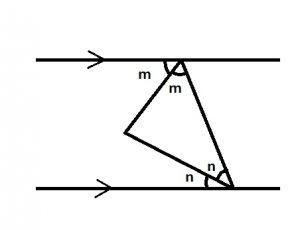Which of the following statements is true from the diagram above?

A. m + n = 90o
B. m = n
C. n > 45o
D. m < 45o

Correct Answer: Option A

A. m + n = 90o